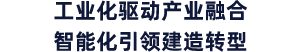展位申请参观登记

• 方案设计
• 初设及施工图设计
• 构件深化设计
• 设计软件
• AEC 软件
• BIM 咨询
• 数据处理系统

• 预制构件成品
• 叠合墙
• 加气混凝土板
• 实心墙
• 三明治墙
• 叠合楼板
• 楼梯
• 阳台

• 钢结构
• 内外墙板
• 楼板
• 屋面板

• 生产流程控制系统
• 混凝土分配穿梭机
• 布料机
• 钢筋铺设机器人
• 振动技术
• 颜色配料系统
• 添加剂计量系统
• 模具清理机
• 喷油机
• 边模机械手
• 绘图机器人
• 摆渡系统
• 码垛机
• 翻转机
• 侧立机
• 水计量及湿度测量装置
• 养护系统
• 混凝土搅拌机
• 成型模具
• 全自动数控弯箍机
• 钢筋桁架焊接生产线
• 全自动钢筋网焊接生产线
• 全自动钢筋矫直切断机
• 数控钢筋弯曲中心

• 预埋件
• 螺栓连接
• 紧固件
• 锚固件
• 阳台连接件
• 夹芯保温墙板连接件
• 支模系统
• 密封胶

• 商品混凝土
• 水泥
• 混凝土添加剂
减水剂、缓凝剂、速凝剂、超塑化剂、固化剂、脱模剂、抗裂剂

• 集成墙面
• 轻质隔墙
• 集成卫浴
• 集成厨房
• 集成地暖
• 集成吊顶
• 全屋定制
• 收纳系统

• 隔热装饰一体化
• 玻璃幕墙
• 节能门窗
• 建筑涂料
• 屋面体系

• 建筑模板
• 模板配件
• 脚手架系统
• 预制构件支撑体系
• 支撑柱
• 安装工具

• 工程车辆
• 土方机械
• 起重和运输设备
• 施工设备、工具和特殊系统
• 混凝土和砂浆在工地的加工和处理
• 工地设施

• 装配式桥梁
• 装配式高架及市政公路
• 地下综合管廊
• 道路施工和养护设备
• 管道和电缆铺设设备和工具

关注我们×

微信关注BIC×